# Deep Learning Best Practices –  Weight Initialization﻿

In this blog I am going to talk about the issues related to initialization of weight matrices and ways to mitigate them. Before that, let’s just cover some basics and notations that we will be using going forward.comments

By Neerja Doshi, University of San Franciscohttps://pixabay.com/photo-1600668/

### Motivation

As a beginner at deep learning, one of the things I realized is that there isn’t much online documentation that covers all the deep learning tricks in one place. There are lots of small best practices, ranging from simple tricks like initializing weights, regularization to slightly complex techniques like cyclic learning rates that can make training and debugging neural nets easier and efficient. This inspired me to write this series of blogs where I will cover as many nuances as I can to make implementing deep learning simpler for you.

While writing this blog, the assumption is that you have a basic idea of how neural networks are trained. An understanding of weights, biases, hidden layers, activations and activation functions will make the content clearer. I would recommend this course if you wish to build a basic foundation of deep learning.

Note — Whenever I refer to layers of a neural network, it implies the layers of a simple neural network, i.e. the fully connected layers. Of course some of the methods I talk about apply to convolutional and recurrent neural networks as well. In this blog I am going to talk about the issues related to initialization of weight matrices and ways to mitigate them. Before that, let’s just cover some basics and notations that we will be using going forward.

### Basics and Notations

Consider an L layer neural network, which has L-1 hidden layers and 1 output layer. The parameters (weights and biases) of the layer l are represented as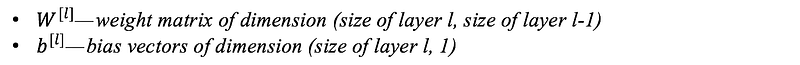In addition to weights and biases, during the training process, following intermediate variables are computed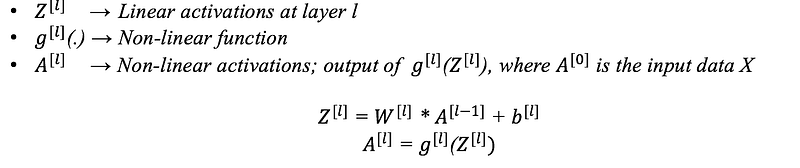Training a neural network consists of 4 steps:

1. Initialize weights and biases.
2. Forward propagation: Using the input X, weights W and biases b, for every layer we compute Z and A. At the final layer, we compute f(A^(L-1))which could be a sigmoid, softmax or linear function of A^(L-1) and this gives the prediction y_hat.
3. Compute the loss function: This is a function of the actual label y and predicted label y_hat. It captures how far off our predictions are from the actual target. Our objective is to minimize this loss function.
4. Backward Propagation: In this step, we calculate the gradients of the loss function f(y, y_hat) with respect to A, W, and b called dA, dW and db. Using these gradients we update the values of the parameters from the last layer to the first.
5. Repeat steps 2–4 for n iterations/epochs till we feel we have minimized the loss function, without overfitting the train data (more on this later!)

Here’s a quick look at steps 2 , 3 and 4 for a network with 2 layers, i.e. one hidden layer. (Note that I haven’t added the bias terms here for simplicity):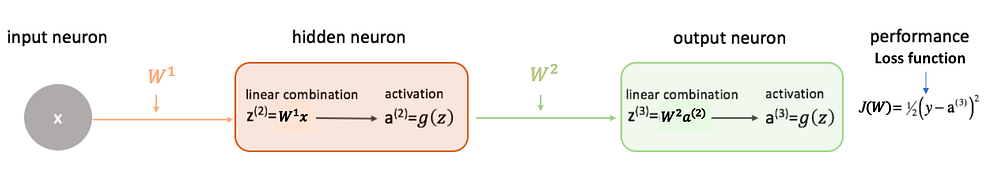Forward Propagation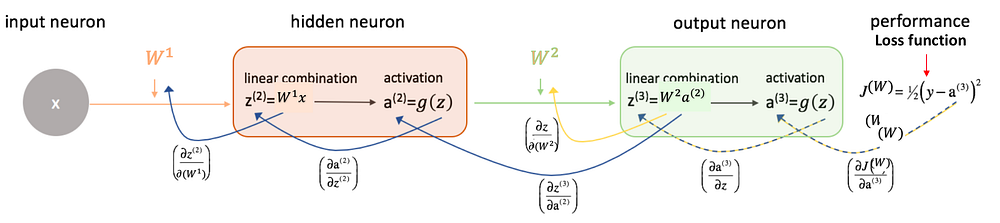Backward Propagation

### Initializing weights W

One of the starting points to take care of while building your network is to initialize your weight matrix correctly. Let us consider 2 scenarios that can cause issues while training the model:

1. Initializing all weights to 0

Let’s just put it out there — this makes your model equivalent to a linear model. When you set all weight to 0, the derivative with respect to loss function is the same for every w in W^l, thus, all the weights have the same values in the subsequent iteration. This makes the hidden units symmetric and continues for all the n iterations you run. Thus setting weights to zero makes your network no better than a linear model. It is important to note that setting biases to 0 will not create any troubles as non zero weights take care of breaking the symmetry and even if bias is 0, the values in every neuron are still different.

2. Initializing weights randomly

Initializing weights randomly, following standard normal distribution (`np.random.randn(size_l, size_l-1) `in Python) while working with a (deep) network can potentially lead to 2 issues — vanishing gradients or exploding gradients.

a) Vanishing gradients — In case of deep networks, for any activation function, abs(dW) will get smaller and smaller as we go backwards with every layer during back propagation. The earlier layers are the slowest to train in such a case.

The weight update is minor and results in slower convergence. This makes the optimization of the loss function slow. In the worst case, this may completely stop the neural network from training further.

More specifically, in case of sigmoid(z) and tanh(z), if your weights are large, then the gradient will be vanishingly small, effectively preventing the weights from changing their value. This is because abs(dW) will increase very slightly or possibly get smaller and smaller every iteration. With RELU(z) vanishing gradients are generally not a problem as the gradient is 0 for negative (and zero) inputs and 1 for positive inputs.

b) Exploding gradients — This is the exact opposite of vanishing gradients. Consider you have non-negative and large weights and small activations A (as can be the case for sigmoid(z)). When these weights are multiplied along the layers, they cause a large change in the cost. Thus, the gradients are also going to be large. This means that the changes in W, by `W — ⍺ * dW,` will be in huge steps, the downward moment will increase.

This may result in oscillating around the minima or even overshooting the optimum again and again and the model will never learn!

Another impact of exploding gradients is that huge values of the gradients may cause number overflow resulting in incorrect computations or introductions of NaN’s. This might also lead to the loss taking the value NaN.

### Best Practices

1. Using RELU/ leaky RELU as the activation function, as it is relatively robust to the vanishing/exploding gradient issue (especially for networks that are not too deep). In the case of leaky RELU’s, they never have 0 gradient. Thus they never die and training continues.

2. For deep networks, we can use a heuristic to initialize the weights depending on the non-linear activation function. Here, instead of drawing from standard normal distribution, we are drawing W from normal distribution with variance k/n, where k depends on the activation function. While these heuristics do not completely solve the exploding/vanishing gradients issue, they help mitigate it to a great extent. The most common are:

a) For RELU(z) — We multiply the randomly generated values of W by: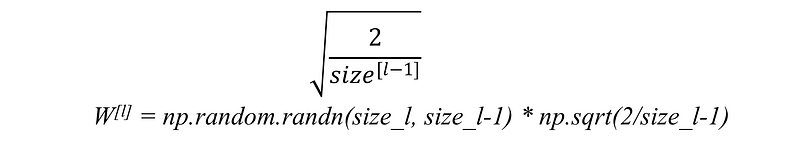b) For tanh(z) — The heuristic is called Xavier initialization. It is similar to the previous one, except that k is 1 instead of 2.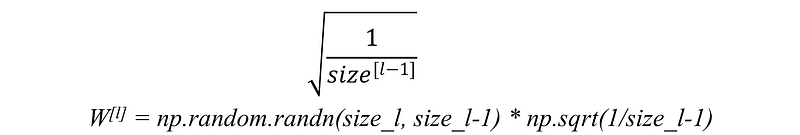In TensorFlow `W = tf.get_variable('W', [dims], initializer)` where `initializer = tf.contrib.layers.xavier_initializer()`

c) Another commonly used heuristic is: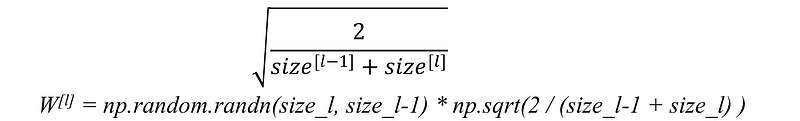These serve as good starting points for initialization and mitigate the chances of exploding or vanishing gradients. They set the weights neither too much bigger that 1, nor too much less than 1. So, the gradients do not vanish or explode too quickly. They help avoid slow convergence, also ensuring that we do not keep oscillating off the minima. There exist other variants of the above, where the main objective again is to minimize the variance of the parameters.

3. Gradient Clipping — This is another way of dealing with the exploding gradient problem. We set a threshold value, and if a chosen function of a gradient is larger than this threshold, we set it to another value. For example, normalize the gradients when the L2 norm exceeds a certain threshold –`W = W * threshold / l2_norm(W) if l2_norm(W) > threshold`

An important point to note is that we have talked about various initializations of W, but not the biases b. This is because the gradients with respect to bias depend only on the linear activation of that layer, and not on the gradients of the deeper layers. Thus there is no diminishing or explosion of gradients for the bias terms. As mentioned earlier, they can be safely initialized to 0.

### Conclusion

In this blog, we’ve covered weight initialization pitfalls and some mitigation techniques. If I have missed any other useful insights related to this topic, I would be happy to learn it from you! In the next blog, I will be talking about regularization methods to reduce overfitting and gradient checking — a trick to make debugging simpler!

References

Bio: Neerja Doshi raduated with MS Data Science at USF and undergrad in Computer Science, and has 2 years of experience in building predictive and recommendation algorithms, and deriving business insights for finance and retail clients. She is excited about opportunities for applying my machine learning and deep learning knowledge to real-world problems. Check out her other blogs here!

Original. Reposted with permission.

Related:

By subscribing you accept KDnuggets Privacy Policy

By subscribing you accept KDnuggets Privacy Policy

By subscribing you accept KDnuggets Privacy Policy